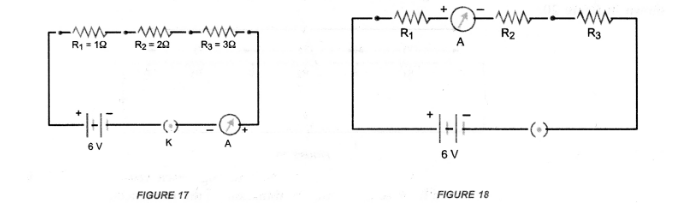# How will you infer with the help of an experiment

Question:

How will you infer with the help of an experiment that the same current flows through every part of the circuit containing three resistances in series connected to a battery ?

Solution:

Perform an activity to show that in series combination of resistors, same current flows through each resistor.

1. Connect three resistors of resistances R1 = 1Ω, R2 = 2Ω and R3 = 3Ω in series. (C.B.S.E. 2014)
2. Connect the series combination of resistors with a battery of 6 V, a plug key K and an ammeter A as shown in figure 17.
3. Note the reading of ammeter after plugging the key. Let it be I.4. Now disconnect ammeter and connect it in between the resistors $R_{1}$ and $R_{2}$ as shown in figure 18 .

5. Again plug the key and note the reading of ammeter. It is found that again it is $I$.

6. Now disconnect the ammeter and connect it in between the resistors $R_{2}$ and $R_{3}$.

7. Plug the key and note the reading of ammeter. Again it is found to be 1 .

Conclusion : Same amount of current flows through each resistor or element connected in series combination.